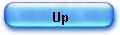HP35 powers of numbers algorithm

To find any power of a positive number, the HP 35 had a key labelled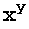which the contrary of what can be found on modern calculators.

This arrangement had been changed as new models arrived (HP-80, introduced in 1973), but it was useful since the machine had no anti-log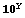function.

More serious was the accuracy problem encountered with the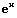function; but that’s another story, I will cover later in details.

Mathematically, the function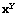is evaluated as: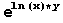and as long the reader has read my previous articles on logarithms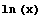and exponentials, the code used by the function is not difficult to understand.

The data is entered as usual for RPN 2 numbers functions as: y ENTER x, for example to calculate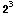, the user has to type 3 ENTER 2 then.

The routine are called internally as if they were typed on the keyboard:

-         X is in C andis called,
-    then Y is flushed from the stack,
-    then finally the exponential routine is called to have the final result.

Let us see the process in details; there again, I provide a commented trace of my examplethat shows only the switches between routines since the standard logarithm, multiplication and exponential code is used (look though how the stack is flushed).

The offset of thefunction (entry point) is 0006, so status bit S2 is set to 1 and control goes to address 02010 which is standard ln(x) routine entry point.

On entry registers status is:

02010: .11...111.                    c -> a[w]
A=02000000000000  B=02999999999999  C=02000000000000
D=03000000000000  M=00000000000000  P=c  S=0.2....78..b

Note that when ln(x) function is called from the keyboard the status bit S9 is set to 1 while in our case it is set to zero. Note also that status bit S8 is set to zero allowing exponential calculation. That will activate later the right railroad switches.

X is in register C normalized formed and we enter the ln(x) function.

At the end of routine the registers are:

02073: 1..1.1.1..                     if s9 = 0
A=06931471805999  B=00000000000000  C=06931471805999
D=03000000000000  M=00000000000000  P=c  S=0.2....7...b

That means ln(2) –our example- is in C and A.

We test Status bit 9 and we go to label “xty22” to flush the stack and get the Y variable in A, before calling routine mpy21 to do the multiplication of  C and A ( ln(x) * y ), using the standard floating point routine.

When the multiplication is done, a test to S8 is made and (S8=0) we go to exponential routine.

That’s all there is to know.

It is almost macro programmation, tough, due to the lack of place, it is not very structured.

J. Laporte
10 January 2006